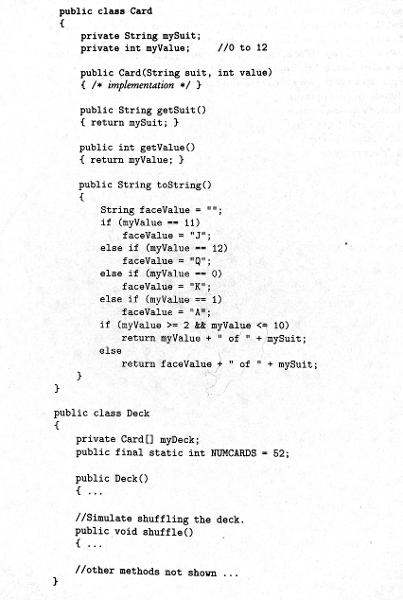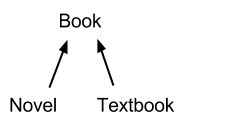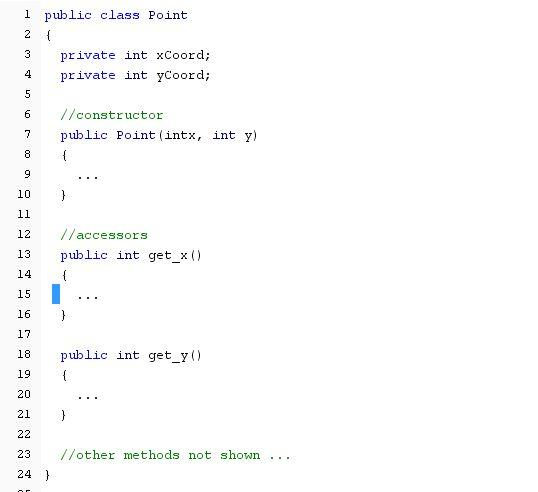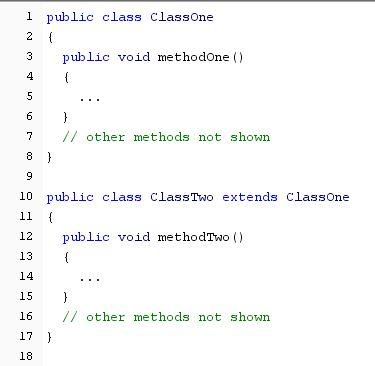# AP COMPSCI FINAL STUDY GUIDE Flashcards

Set Details Share
created 7 years ago by BuzzRiceyear
1,880 views
updated 7 years ago by BuzzRiceyear
Subjects:
computer science
Page to share:
Embed this setcancel
COPY
code changes based on your size selection
Size:
X

1

Refer to the following method.

public static int mystery(int n)
{
if (n == 1)
return 3;
else
return 3 * mystery(n - 1);
}

What value does mystery(1) return?

3

2

If a, b, and c are integers, which of the following conditions is sufficient to guarantee that the expression

a < c || a < b && !(a == c)

evaluates to true?

a < c

3

When will the result be equal to 3?

public static int whatIsIt(int x, int y)
{
int result = 0;
if (x > y)
result = 4;
else
result = 3;

return result;
}

x <= y

4

What is the output of the following Java code?

int x = 0;
if (x < 0)
System.out.print("One ");
System.out.print("Two ");
System.out.print("Three");

Two Three

5

Consider the method
public String mystery(String s)
{
String s1 = s.substring(0,1);
String s2 = s.substring(1, s.length() - 1);
String s3 = s.substring(s.length() - 1);

if (s.length() <= 3)
return s3 + s2 + s1;

else
return s1 + s2 + s3;
}

What is the output of
System.out.println(mystery("DELIVER"));

DELIVER

6

1. Suppose x = 2 and y = 3. If the statement x *= y; is executed once, what is the value of x?

A. 2
B. 3
C. 5
D. 6

D

7

2. Based on the code below:

String sentence;
String str1, str2, str3;
int length1, length2;

sentence = "Today is Wednesday.";

str1 = sentence.substring(9, 18);
str2 = str1.substring(0, 3);
str3 = sentence.replace('d', '*');

length1 = sentence.length();
length2 = str1.length();

System.out.print(str3);

A. To*ay is We*nes*ay.
B. Today * Wednesday
C. Today is Wednesday
D. **d** ** **d***d**

A

8

3. Consider the following method definition:

public static void printTopHalf()
{
}

The word static :

A. is called the return type
B. is called the method name
C. surrounds the parameter list
D. is needed if the method is called from main()
E. surrounds the method body
F. is known as the access specifier

D

9

4. When you define the procedure, create a ______________ for each value you want to pass into the procedure.

A. instance variable
B. local variable
C. formal parameter
D. actual parameter

C

10

5. Look at the following use of the substring() method:

String word = "Hello World!";
String subs = word.substring(1,2);

The String subs will contain:

A. "H"
B. "He"
C. "el"
D. "e"

D

11

6. When defining a function, (unlike a procedure), you must specify a ____________.

A. return statement
B. access specifier
C. parameter list
D. return type

D

12

7. Consider the following code segment:

if (n != 0 && x / n > 100)
statement1;
else
statement2;

If n is of type int and has a value of 0 when the segment is executed, what will happen?

A. An ArithmeticException will be thrown.
B. A syntax error will occur.
C. statement1, but not statement2, will execute.
D. statement2, but not statement1, will execute.
E. Neither statement1 nor statement2 will be executed; control will pass to the first statement following the if statement.

D

13

8. Given two strings, a and b, return the result of putting them together in the order abba, e.g. "Hi" and "Bye" returns "HiByeByeHi".

|| EXAMPLES:
|| makeAbba("Hi", "Bye") → "HiByeByeHi"
|| makeAbba("Yo", "Alice") → "YoAliceAliceYo"
|| makeAbba("What", "Up") → "WhatUpUpWhat"

public String makeAbba(String a, String b)
{
String result = "";
result = _________________

return result;
}

What would result need to be in order to finish this function?

A. a + b + b +a;
B. "Hello " + name + "!";
C. first + last + last + first;
D. b + a + a + b;
E. last + first + first + last;

A

14

The elements inside the class body are surrounded with, or delimited by:
A braces{ }
B parenthesis ( )
C quotations " "
D brackets [ ]

A

15

In a main method definition, the symbols appearing inside the ( ) are called the:

A. getters
B. parameter list
C. methods
D. setters

B

16

Which of these words that can appear in a Java program are not a reserved word? *Hint: It does not show up in blue text in Dr. Java.
A. int
B. out
C. class
D. void

B

17

Here is a portion of the source code for a computer program:
69 6D 70 6F 72 74 20 6A-61 76 61 2E 61 77 74 2E 3B 0D 0A 69 6D 70 6F-72 74 20 6A 61 76 61 2E 61 77 74 2E 65 6E-74 2E 2A 3B 0D 69 6D

This program is an example of _________________.

B. machine language
C. HLL
D. assembly language

B

18

True or False: The following statement, written in the interactions pane, will compile and print to the console.

System.out.println("said Sally. "I've said so." See?);

False

19

Which of these must appear outside the body of a class?

A. variable definitions
B. instance variables
C. import statements
D. constructors

Response Feedback: The import statement cannot go inside the body of the class. Output statements must appear in a method. Comments can appear anywhere. Variable definitions can go inside a method or inside a class.

C

20

Which of these is a legal (that is, syntactically correct) Java class name?

A. U2
B. 2U
C. U-2
D. 2_U

Response Feedback: While letters, digits and underscores are legal in identifiers, you cannot start with a digit. You also cannot have spaces or hyphens in an identifier. That leaves U2.

A

21

Write the main method heading: It must be exactly as Java needs it to be for your code to compile.

Hint: *No hint this time.

public static void main (String[] args)
public static void main(String[ ] args)
public static void main(String[] args)
public static void main (String[ ] args)

public static void main (String[] args)
public static void main(String[ ] args)
public static void main(String[] args)
public static void main (String[ ] args)

22

The following line of code is going to print out what type of value?

System.out.print("575");

A. double
B. int
C. String

C

23

What is the output of the following code segment?

int myAge = 63;

int kathysAge = myAge;

kathysAge = 60;

System.out.print(myAge);

A. 63
B. myAge
C. 60
D. kathysAge

A

24

1. Evaluate the following expression. Make sure that your answer is correct type. For instance, if the answer is 2 and the type is int, then you should enter 2. If the answer is 2, and the type is double, then enter 2.0.
13 + Math.abs(-7) - Math.pow(2.0, 3) + 4

16.0

25

2. Evaluate the result from evaluating the following integer expression. Make sure that your answer is the correct type. For instance, if the answer is 2 and the type is int, then you should enter 2. If the answer is 2, and the type is double, then enter 2.0.
26 % 10 % 4 * 2

4

26

3. In the assignment statement below, what is the purpose of the (double) ?
int x = 3, y = 2;
double z = (double) x / y;

A. To double the amount of the answer, like multiplying by 2.
B. To make sure that the result of the division is a decimal number.
C. To round the answer to the nearest integer.
D. To round the answer to the nearest tenth.

B

27

4. Which of the following code fragments will cause an error?
A. String greeting = "Hello, Kevin!";
System.out.print(greeting);
B. int luckyNumber;
System.out.println(luckyNumber);
C. System.out.println("Hi " + 5);

B

28

5. The statement System.out.print ("No" + (2 + 3) + "way"); produces the output:
A. No5way
B. No 2 3 way
C. No23way
D. None. Illegal statement

A

29

6. In Java, Scanner is a class in which package?
A. java.lang
B. java.text
C. java.util
D. java.io

C

30

7. Evaluate the result from evaluating the following integer expression. Make sure that your answer is the correct type. For instance, if the answer is 2 and the type is int, then you should enter 2. If the answer is 2, and the type is double, then enter 2.0.
(12 + 4) / 4 * 2

8

31

8. Which of the following statements correctly creates a Scanner object for keyboard input?
A. Keyboard scanner = new Keyboard(System.in);
B. Scanner keyboard = new Scanner(System.in);
C. Scanner cin = new Scanner(System.Keyboard);
D. Scanner keyboard(System.in);

B

32

9. Which of the following is not a primitive data type?
A. int
B. String
C. double

B

33

10. What's the result of the following code snippet?
public static void main(String[ ] args)
{
double bottles;
double bottles_volume = bottles * 2;
System.out.println(bottle_volume);
}
A. 0
B. 1
C. 2
D. compile-time error

D

34

1. Refer to the following code fragment:

double answer = 12 / 7;
System.out.println("12 / 7 = " + answer);

The output is

12 / 7 = 1

The programmer intends the output to be

12 / 7 = 1.7142857142857142

Which of the following replacements for the first line of code will not fix the problem?

A. double answer = 12.0 / 7;
B. double answer = 12 / 7.0;
C. double answer = 12.0 / 7.0;
D. int answer = (12 / 7);

D

35

2. Which one of the following is a correct method of declaring and initializing an double variable with name crossett?
A. crossett = 30;
B. crossett = double 30;
C. double crossett = 30;
D. double crossett;

C

36

3. The statement System.out.print("No" + "2 + 3" + "way"); produces the output:
A. No5way
B. No2 + 3way
C. NoNowaywayway
D. No+6+way

B

37

4. What value is stored in result if:

int result = 10 - 2 % 2;

A. 10
B. 1
C. 4
D. 0

A

38

5. True or False: All of the following statements are true.
In Java, 12 % 5 and 12 / 5 have the same answer.
The names int and double describe the type of variable being initialized or declared.

True

39

6. Which of the following variable names is an example of a constant?
A. milesPerHour
B. MILES_PER_HOUR
C. MilesPerHour
D. final

B

40

7. Refer to the following code fragment below:

public String yourName = "Kevin";

The name yourName refers to:
A. the type of variable
B. it's ability to be used by other methods.
C. the name of the variable.
D. the value of the variable

C

41

8. Define: Define Concatenation operator.

+

42

9. What is the name of the following Scanner object?
Scanner cin = new Scanner(System.in);

cin

43

10. In a variable definition, the kind of value stored inside is called its:
A. method
B. type
C. class
D. assignment

B

44

When a Java string does not have surrounding double quotes, what type of error occurs?

Compile-time error

45

Text, like everything else in your computer, is stored as raw binary numbers. To treat those numbers as characters, they must be encoded. The encoding scheme used in Java is called:

Unicode

46

Programs that are designed to "work for you", carrying out a task you specify, are known as

application programs

47

The devices that feed data and programs into computers are called ______.

Input Devices

48

If your java source code program compiles, but displays an error message when you run it, then it must have a:

runtime error

49

What is the computer component that stores program instructions during a program execution?

memory

50

Four common predefined escape sequences
\t - Tab (numeric value 9, Ctrl+I or Tab)
\n - Newline (numeric value 10, Ctrl+J)

Place a double quote inside a string:
System.out.print("then she said, \"Hello\"");

Place a backslash inside a string
System.out.println("C:\\Windows\\my.ini");

Know the slide 29 on Week 1.2.

51

Each memory cell has a unique location in main memory, called the _________.

52

Which of the following will correctly check to see if the variable x is equal to 5?

if (x == 5)

53

What is the output of the following code snippet, if the input is 25?

public static void main(String[ ] args)

{

Scanner cin = new Scanner(System.in);

int i = cin.nextInt( );

if(i > 24)

{

i++;

}

else

{

i--;

}

System.out.println(i);

}

26

54

What is the output of the following Java code?

int x = 0;

if(x < 1)

System.out.print(“One “);

System.out.print(“Two “);

System.out.print(“Three”);

One Two Three

55

What is the value of the price variable after the following code snippet is executed?

int price = 42;

if(price < 45)

{

price = price + 10;

}

if(price < 30)

{

price = price * 2;

}

if(price < 100)

price = price - 20;

}

32

56

What is the output of the following code snippet?

public static void main(String[ ] args)

{

int num = 100;

if(num != 100)

{

System.out.println(“Not 100”);

}

else

{

System.out.println(“100”);

}

}

100

57

What is the output of the following code snippet?

public static void main(String[ ] args)

{

double income = 45000;

double cutoff = 55000;

double min_income = 30000;

if(min_income > income)

{

System.out.println(“Minimum income requirements not met.”);

}

if(cutoff < income)

{

System.out.println(“Income requirement is met.”);

}

else

{

System.out.println(“Maximum income limit is exceeded.”);

}

}

Maximum income limit is exceeded.

58

What is the output of the following code snippet?

public static void main(String[ ] args)

{

int s1 = 20;

if(s1 < 20)

{

System.out.print(“1”);

}

if(s1 <= 40)

{

System.out.print(“2”);

}

if(s1 > 20)

{

System.out.print(“3”);

}

}

2

59

What is the syntax error in the following method definition?

public static area(double r)

{

double a;

a = 3.14 * r * r;

return r * r;

}

The method does not specify a return type.

60

When you try to convert String data to numeric values, using the Integer.parseInt() or Double.parseDouble() functions on a String that is improperly formatted (containing spaces, commas, or other illegal characters) you will get:

a NumberFormatException

61

Consider the following method definition:

public static void printTopHalf()

{

}

The word printTopHalf :

is called the method name

62

If you use the String searching functions to search for a particular character or substring, and the value you’re searching for is not found, the methods will return:

-1

63Which of the following represents correct /* implementation */ code for the constructor in the card class?

mySuit = suit;

myValue = value;

641. Consider this hierarchy, in which Novel and Textbook are subclasses of Book.

Which of the following is a true statement about the classes shown?

Each of the classes - Book, Novel, and Textbook - can have a method computeShelfLife, whose code in Book and Novel is identical, but different from the code in Textbook.

65

A programmer is designing a program to catalog all books in a library. She plans to have a Book class that stores features of each book: author, title, isOnShelf, and so on, with operations like getAuthor, getTitle, getShelfInfo, and setShelfInfo. Another class, LibraryList, will store an array of Book objects. The LibraryList class will include operations such as listAllBooks, addBook, removeBook, and searchForBook. The programmer plans to implement and test the Book class first, before implementing the LibraryList class. The programmer’s plan to write the Book class first is not an example of

top-down development.

66The perimeter and area methods are abstract because there’s no suitable default code for them.

67

A programmer plans to write a program that simulates a small bingo game (no more than six players). Each player will have a bingo card with 20 numbers from 0 to 90 (no duplicates). Someone will call out numbers one at a time, and each player will cross out a number on his card as it is called. The first player with all the numbers crossed out is the winner. In the simulation, as the game is in progress, each player’s card is displayed on the screen.

The programmer envisions a short driver class (sometimes called test class) whose main method has just two statements:

BingoGame b = new BingoGame( );

b.playBingo( );

The BingoGame class will have several objects: a Display, a Caller, and a PlayerGroup. The PlayerGroup will have a list of Players, and each Player will have a BingoCard.

The relationship between the PlayerGroup and Player classes is an example of

composition

68The method getLabels is wrong and should be getLabels().

69Which represents correct /* implementation code */ for the Rectangle constructor?

I super(labels, topLeft, botRight);

II super(labels);

this.myTopLeft = topLeft;

this.myBotRight = botRight;

III super(labels);

myTopLeft = topLeft;

myBotRight = botRight;

II and III only

70Consider an ArrayList<Quadrilateral> quadList whose elements are of type Rectangle, Parallelogram, or Square.

Refer to the following method, writeAreas:

What is the effect of executing this method?

The appropriate area method for each quad in quadList will be determined.

71Refer to the definitions of ClassOne and ClassTwo below.

Consider the following declarations in a client class. You may assume that ClassOne and ClassTwo have default constructors.

ClassOne c1 = new ClassOne();

ClassTwo c2 = new ClassTwo();

Which of the following method calls will cause an error?

I. c1.methodTwo();

II. c2.methodTwo();

III. c2.methodOne();

I only

72

Which of the following will execute without throwing an exception?

I. String s = null;

String t = "";

if (s.equals(t))

System.out.println("empty strings?");

II. String s = "holy";

String t = "moly";

if (s.equals(t))

System.out.println("holy moly!");

III. String s = "holy"

String t = s.substring(5);

System.out.println(s + t);

II only

73

A programmer plans to write a program that simulates a small bingo game (no more than six players). Each player will call out numbers one at a time, and each player will cross out a number on his card at it is called. The first player with all the numbers crossed out is the winner. In the simulation, as the game is in progress, each player's card is displayed on the screen.

The programmer envisions a short driver class whose main method has just two statements:

BingoGame b = new BingoGame();

b.playBingo();

The BingoGame class will have several objects: a display, a Caller, and a PlayerGroup.

The PlayerGroup will have a list of Players, and each Player will have a BingoCard.

The relationship between the PlayerGroup and Player classes is an example of

composition.

74

Which of the following correctly initializes an array arr to contain four elements each with value 0?

I. int[] arr = {0, 0, 0, 0};

II int[] arr = new int;

III int[] arr = new int ;

for (int i = 0; i < arr.length; i++)

arr[i] = 0;

I and III only

75

Consider the following method.

public static int mystery(int[] arr)

{

int x = 0;

for (int k = 0; k < arr.length; k = k + 3)

x = x + arr[k];

return x;

}

Assume that the array nums has been declared and initialized as follows.

int[] nums = {3, 6, 1, 0, 4, 1, 2};

What value will be returned as a result of the call mystery(nums) ?

5

76

Consider the following method.

public ArrayList<Integer> mystery(int n)

{

ArrayList<Integer> seq = new ArrayList<Integer>();

for (int k = 0; k <= n; k++)

seq.add(new Integer(k * k + 3));

return seq;

}

Which of the following is printed as a result of executing the following statement?

System.out.println(mystery(6));

[3, 4, 7, 12, 19, 28, 39]

77

Consider the following code segment.

int x = 7;

double y = 3;

if ((x < 10) && (y < 0))

System.out.println("Value is: " + x * y);

else

System.out.println("Value is: " + x / y);

What is printed as a result of executing the code segment?

Value is: 2.3333333